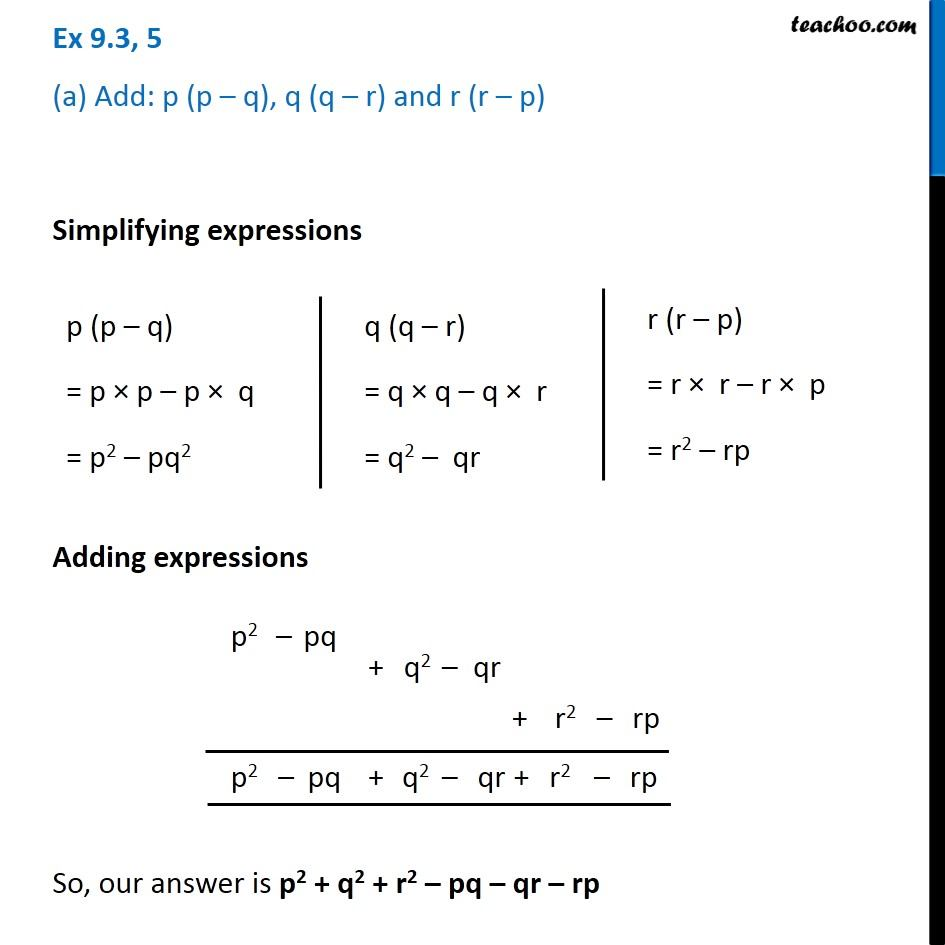Multiplication of Monomials by Binomials and Trinomials

Chapter 8 Class 8 Algebraic Expressions and Identities
Concept wiseLearn in your speed, with individual attention - Teachoo Maths 1-on-1 Class

### Transcript

Ex 8.3, 5 (a) Add: p (p – q), q (q – r) and r (r – p) Simplifying expressions p (p – q) = p × p – p × q = p2 – pq2 q (q – r) = q × q – q × r = q2 – qr r (r – p) = r × r – r × p = r2 – rp So, our answer is p2 + q2 + r2 – pq – qr – rp